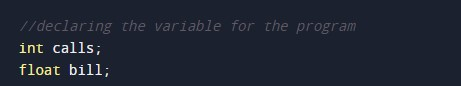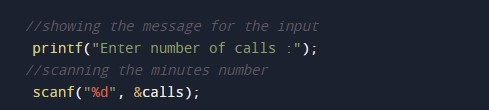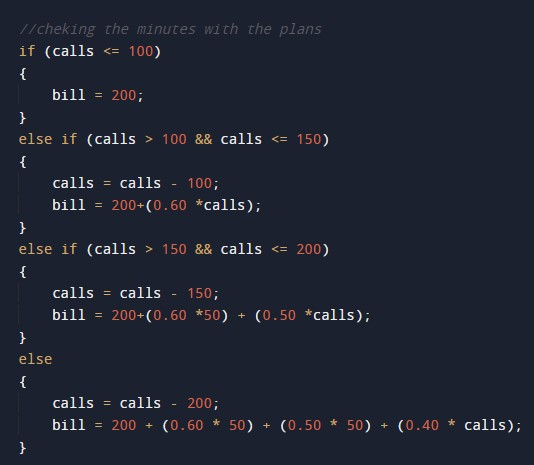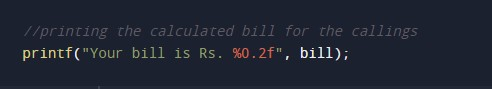C Program to Calculate Telephone Call Bills

In this tutorial you will learn about the C Program to Calculate Telephone Call Bills and its application with practical example.

C Program to Calculate Telephone Call Bills

In this tutorial, we will learn to create a C program that will Calculate Telephone Call Bills using C programming.

Prerequisites

Before starting with this tutorial we assume that you are best aware of the following C programming topics:

• Operators in C Programming.
• Basic Input and Output function in C Programming.
• Basic C programming.
• While loop in C programming.
• Arithmetic operations in C Programming.

Program to Calculate Telephone Call Bills

In c programming, it is possible to take numerical input from the user and calculate Telephone Call Bills with the help of a very small amount of code. The C language has many types of header libraries which has supported function in them with the help of these files the programming is easy. But today we will Calculate Telephone Call Bills using c programming. As per the following rule:

Minimum Rs. 200 for up to 100 calls.
Plus Rs. 0.60 per call for the next 50 calls.
Plus Rs. 0.50 per call for the next 50 calls.
Plus Rs. 0.40 per call for any call beyond 200 calls.

With the help of this program, we can Calculate Telephone Call Bills.

Output:-In the above program, we have first initialized the required variable.• calls = it will hold the integer value for the calls in minutes.
• bill = it will hold the floating value for the bills.

Input number from the user.Program Logic Code.Printing output for bill generated.In this tutorial we have learn about the C Program to Calculate Telephone Call Bills and its application with practical example. I hope you will like this tutorial.